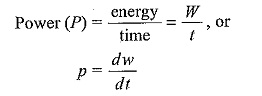# Power and Energy

## Power and Energy:

Power and Energy – Power is the rate of change of energy, and is denoted by either P or p. If certain amount of energy is used over a certain length of time, thenwhere dw is the change in energy and dt is the change in time.

We can also writeEnergy is measured in joules (J), time in seconds (s), and power in watts (W).

By definition, one watt is the amount of power generated when on joule of energy is consumed in one second. Thus, the number of joules consumed in one second is always equal to the number of watts. Amounts of power less than one watt are usually expressed in fraction of watts in the field of electronics; viz. milliwatts (mW) and microwatts (μW). In the electrical field, kilowatts (kW) and megawatts (MW) are common units. Radio and television stations also use large amounts of power to transmit signals.

Updated: September 29, 2017 — 9:31 pm

`Please enable JavaScript in your browser to complete this form.Name *FirstLastEmail *Paragraph TextName`
` `